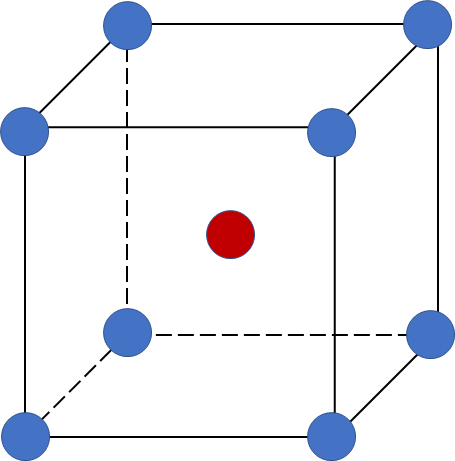# Problem: Barium crystallizes in a body-centered cubic unit cell with an edge length of 5.025 Å(b) Calculate the density of barium.

97% (64 ratings)
###### FREE Expert Solution

We’re being asked to calculate the density of barium that crystallizes in a body-centered cubic unit cell.

A body-centered cubic (BCC) unit cell is composed of a cube with one atom at each of its corners and one atom at the center of the cubeRecall that density has a formula of:

97% (64 ratings)###### Problem Details

Barium crystallizes in a body-centered cubic unit cell with an edge length of 5.025 Å

(b) Calculate the density of barium.

Frequently Asked Questions

What scientific concept do you need to know in order to solve this problem?

Our tutors have indicated that to solve this problem you will need to apply the Unit Cell concept. You can view video lessons to learn Unit Cell. Or if you need more Unit Cell practice, you can also practice Unit Cell practice problems.

What is the difficulty of this problem?

Our tutors rated the difficulty ofBarium crystallizes in a body-centered cubic unit cell with ...as medium difficulty.

How long does this problem take to solve?

Our expert Chemistry tutor, Sabrina took 7 minutes and 49 seconds to solve this problem. You can follow their steps in the video explanation above.

What professor is this problem relevant for?

Based on our data, we think this problem is relevant for Professor Tang's class at USF.

What textbook is this problem found in?

Our data indicates that this problem or a close variation was asked in Chemistry - OpenStax 2015th Edition. You can also practice Chemistry - OpenStax 2015th Edition practice problems.